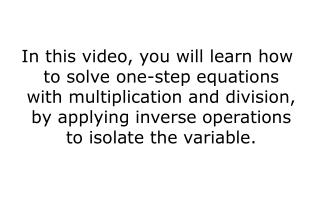# In this video, you will learn how to solve one-step equations with multiplication and division, by applying inverse oper - PowerPoint PPT PresentationDownload PresentationIn this video, you will learn how to solve one-step equations with multiplication and division, by applying inverse oper

In this video, you will learn how to solve one-step equations with multiplication and division, by applying inverse operDownload Presentation## In this video, you will learn how to solve one-step equations with multiplication and division, by applying inverse oper

- - - - - - - - - - - - - - - - - - - - - - - - - - - E N D - - - - - - - - - - - - - - - - - - - - - - - - - - -
##### Presentation Transcript

1. In this video, you will learn how to solve one-step equations with multiplication and division, by applying inverse operations to isolate the variable.

2. equation – a mathematical statement that two expressions are equal example: 4 x 5 = 2 x 10

3. equation – a mathematical statement that two expressions are equal example: 4 x 5 = 2 x 10

4. inverse operation – any operation that undoes another operation • addition and subtraction • multiplication and division

5. inverse operation – any operation that undoes another operation • addition and subtraction • multiplication and division

6. inverse operation – any operation that undoes another operation • addition and subtraction • multiplication and division

7. When a fraction has a numerator and a denominator of the same value, the fraction is equal to 1.

8. 1

9. 1

10. 1. Determine which operation will help you isolate the variable. • 2. Apply the operation to both sides of the equation. • 3. Verify that you are correct by substituting your solution for the variable. Solving a One-Step Equation(Goal: Get the variable by itself.)

11. 1. Determine which operation will help you isolate the variable. • 2. Apply the operation to both sides of the equation. • 3. Verify that you are correct by substituting your solution for the variable. Solving a One-Step Equation(Goal: Get the variable by itself.)

12. 1. Determine which operation will help you isolate the variable. • 2. Apply the operation to both sides of the equation. • 3. Verify that you are correct by substituting your solution for the variable. Solving a One-Step Equation(Goal: Get the variable by itself.)

13. 40 = 5z Solve the equation to find the value of z.

14. 40 = 5z Prove that your answer is correct. (Verify that z = 8.)

15. = 2 Solve the equation to find the value of n.

16. = 2 Prove that your answer is correct. (Verify that n = 12.)

17. In this video, you learned how to solve one-step equations with multiplication and division, by applying inverse operations to isolate the variable.